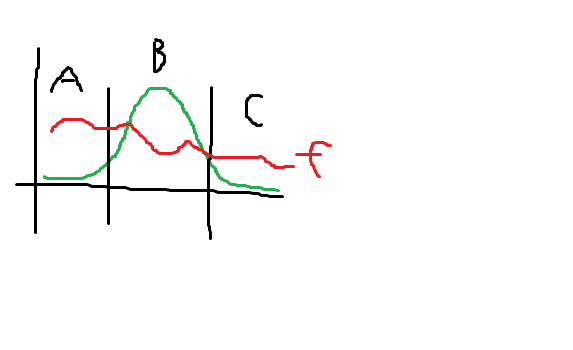Convergence of the Expected Value of a Function

Suppose that nx is binomially distributed: B((n-1)p, (n-1)p(1-p))
I wish to find the expected value of a function f(x), thus
$\sum_{nx=0}^{n-1} B() f(x)$
Assume that f() is non-linear, decreasing and continuous, f(x) = c is [0,1] to [0, ∞)
I want to show that the above sum converges to f(p) if n→∞
By computation using all types of different functions (even very extreme ones), it is clear that it converges to that. The only thing is that while the proof looks like it'd be simple, I just couldn't figure it out. If f() is linear, then we just get f((n-1)p/n) which converges to f(p), if it's not, Jensen's inequality tells us that we will get something else.

If we are not happy with the sum we can use n→∞ to show the sum goes to:
$$\int_{0}^{∞} \text{(normal distribution of nx with same mean and variance as above)} f(nx/n) dnx$$

Just wondering if anyone has a tip or so of how I can approach this. Thanks

Stephen Tashi
Suppose that nx is binomially distributed: B((n-1)p, (n-1)p(1-p))
I'm used to seeing the binomial written as B(n,p) or, in your case, it woud be B(n-1,p). I think you have given the parameters as the mean and variance instead of the number of trials and the probability of succes.

I wish to find the expected value of a function f(x), thus
$\sum_{nx=0}^{n-1} B() f(x)$

It is completely unclear what you mean by that.

Are you treating "nx" as one variable or is it the product (n)(x)?

What is the argument of the function B() ? B(x)? B(nx)? B(n)?

Are you trying to use the density of the binomial distribution:
$b(k,n,p) = C^n_k p^k (1-p)^{n-k}$ ?
or, in your case $B(k) = b(k,n-1,p)$.

I am trying to valuate the following sum:

$$\sum_{i=0}^{n-1} C_i^{n-1} \; p^i (1-p)^{n-1-i} f(\frac{i}{n}) = c$$

where c is a real, positive constant, f is a decreasing function, and I am trying to evaluate p. I know that the end result when n → ∞ is $p = f^{-1} (c)$ and I want to prove it.

I previously defined $x= \frac{i}{n}$ (thus i = xn) to make f() independent of n, which allows me to take the limit of n and converge the sum into an integral using demoivre-laplace theorem.

And sorry, by binomial I did mean b(n-1, p). Hope this makes it clearer

So rephrasing, you want to find:
$$V = lim_{n \rightarrow \infty} \sum_{i=0}^{n} {{n}\choose{i}} p^i (1-p)^{n-i} f(\frac{i}{n})$$

Our goal is now to pick an interval around the mean np, which includes an increasing number of standard deviations around the mean as n goes to infinity (which means the interval covers an amount of probability that goes to 1), but where the interval also becomes a smaller proportion of n as n goes to infinity (which means the interval includes only values from a small local part of f). Then we can show that the sum can be approximated only by values from within this interval, and provide converging upper and lower bounds for the sum within the interval.

Here's a picture of the interval (labeled B) and the parts beside it (A and C):The variance of the binomial distribution is np(1-p), for a standard deviation of $\sqrt{np(1-p)} = \sqrt{n} \sqrt{p(1-p)}$. So the size of the interval must grow faster than $\sqrt{n} \sqrt{p(1-p)}$ in order to include an increasing number of standard deviations around the mean. It must also grow slower than n, in order to decrease relative to n. So by picking any function asymptotically between $sqrt{n}$ and n, let's choose the width of the interval to be $2 n^{2/3}$. We want the interval to be centered about the mean, and since it's used in the sum the endpoints must be integers, so that gives us $(\lceil np - n^{2/3} \rceil, \lceil np + n^{2/3} \rceil)$

Now rewrite the limit in terms of the parts of the sum outside the interval, and inside the interval:
$$lim_{n \rightarrow \infty} \left( \sum_{i=0}^{\lceil{np-n^{2/3}}\rceil} {{n}\choose{i}} p^i (1-p)^{n-i} f(\frac{i}{n}) + \sum_{i=\lceil{np-n^{2/3}}\rceil + 1}^{\lceil{np + n^{2/3}}\rceil} {{n}\choose{i}} p^i (1-p)^{n-i} f(\frac{i}{n}) + \sum_{i=\lceil{np + n^{2/3}}\rceil + 1}^{n} {{n}\choose{i}} p^i (1-p)^{n-i} f(\frac{i}{n}) \right)$$
And let's label those three parts of the limit as follows, to refer to them:
$$lim_{n \rightarrow \infty} \left( A(n) + B(n) + C(n) \right)$$

The total amounts of probability contained within A, B, and C are:
$$A_p(n) = \sum_{i=0}^{\lceil{np-n^{2/3}}\rceil} {{n}\choose{i}} p^i (1-p)^{n-i}$$
$$B_p(n) = \sum_{i=\lceil{np-n^{2/3}}\rceil + 1}^{\lceil{np + n^{2/3}}\rceil} {{n}\choose{i}} p^i (1-p)^{n-i}$$
$$C_p(n) = \sum_{i=\lceil{np + n^{2/3}}\rceil + 1}^{n} {{n}\choose{i}} p^i (1-p)^{n-i}$$

Also, denote the maximum and minimum values for f within A, B, and C by $A_{fmin}(n), B_{fmin}(n), C_{fmin}(n), A_{fmax}(n), B_{fmax}(n) C_{fmax}(n)$.

Now it's in a solvable form. To solve it, first find a lower bound on the limit, by finding lower bounds on A, B, and C. A is lower bounded by $A_{fmin}(n) A_p(n)$. And similarly for B and C.
$$L = lim_{n \rightarrow \infty} ( A_{fmin}(n) A_p(n) + B_{fmin}(n) B_p(n) + C_{fmin}(n) C_p(n))$$
Also find an upper bound:
$$U = lim_{n \rightarrow \infty} ( A_{fmax}(n) A_p(n) + B_{fmax}(n) B_p(n) + C_{fmax}(n) C_p(n))$$

Now you must prove that L = f(p). First, since $A_p(n)$ and $B_p(n)$ go to 0 as n increases, the first and third parts of the sum go to 0. Second, $B_p(n)$ goes to 1 as n increases (due to careful choice of the interval, earlier). And third, f is continuous, so $B_{fmin}$ approaches f(p) as the interval shrinks around p.

In a similar fashion you prove that U = f(p). Then since the limits in U and L sandwich V from above and below, you can conclude V = f(p).